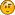###Author Topic: Calculate rotation angle from movement (translation)  (Read 1854 times)

####K24A3

• long
•• Posts: 231##### Calculate rotation angle from movement (translation)
« on: October 09, 2011, 10:58:07 am »
I'm trying to rotate an object so it's facing the way it's moving.

Is there a function in jPCT or Java that can calculate the rotation angle based on an object's translation movement?

If the object is travelling up Z using translation(0,0,2), the angle would be 270 degrees.
If the object is travelling down Z  using translation(0,0,-2), the angle would be 90 degrees (or PI/2).
If the object is travelling up X  using translation(2,0,0), the angle would be 0 degrees.
If the object is travelling down X  using translation(-2,0,0), the angle would be 180 degrees (or PI).
If the object is travelling down X and up Z using translation(-2,0,2), the angle would be 225 (270-180).

####EgonOlsen

••• Posts: 12035##### Re: Calculate rotation angle from movement (translation)
« Reply #1 on: October 09, 2011, 01:40:55 pm »
You can calculate the direction vector from the old position to the new one, call one of the getRotationMatrix()-methods on the resulting SimpleVector and use that as the new rotation matrix of yout object. Working with derived angles from a matrix or something usually is a bad idea.

####K24A3

• long
•• Posts: 231##### Re: Calculate rotation angle from movement (translation)
« Reply #2 on: October 09, 2011, 02:10:10 pm »
The problem is that the destination vector is unknown since the object is moved on the fly or by random.

I created an algorithm myself which works fine, I was hoping there was a pre-existing function to do the math.

Here's the code if anyone needs it for something.
Code: [Select]
` float fCombined = 0; if(xMove  < 0) fCombined = -xMove; else           fCombined =  xMove; if(zMove  < 0) fCombined += -zMove; else           fCombined +=  zMove; float fAngle = fPI; if(zMove > 0) fAngle = fPIhalf*3; else          fAngle = fPIhalf*1; if(zMove > 0) // 270 { if(xMove > 0) fAngle += (xMove/fCombined) * fPI/2; else          fAngle -= (-xMove/fCombined) * fPI/2; } else // 90 { if(xMove > 0) fAngle -= (xMove/fCombined) * fPI/2; else          fAngle += (-xMove/fCombined) * fPI/2; }                                myobject.clearRotation();                                myobject.RotateY(fAngle)`

####EgonOlsen

••• Posts: 12035##### Re: Calculate rotation angle from movement (translation)
« Reply #3 on: October 09, 2011, 02:12:05 pm »But once you know the translation, you know the destination....i don't get the point here. Anyway, if you have something that works for you, all is well...

####K24A3

• long
•• Posts: 231##### Re: Calculate rotation angle from movement (translation)
« Reply #4 on: October 09, 2011, 02:42:24 pm »
Hmm I guess I could place the Move variables into a simpleVector then scaleMul it to generate a destination. I'll use that technique if I need Y movement